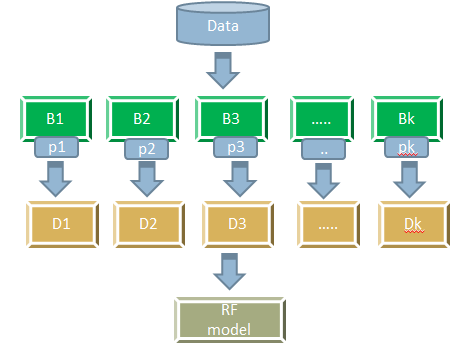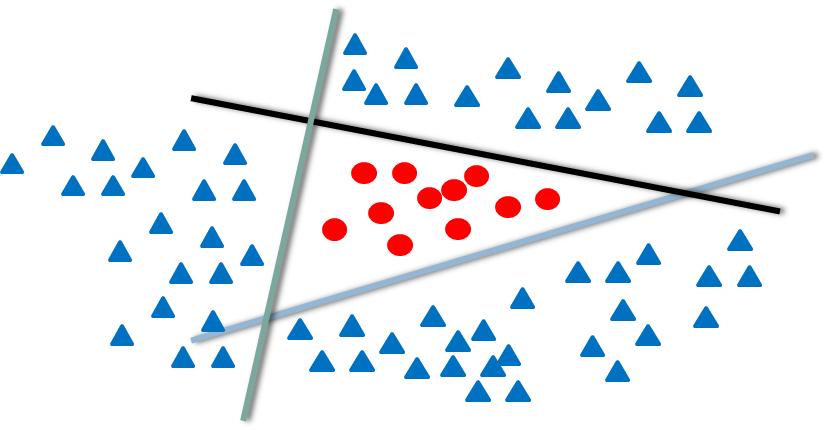• No products in the cart.

# 203.7.5 The Random Forest

### Random Forest

In previous section, we studied about The Bagging Algorithm

• Like many trees form a forest, many decision tree model together form a Random Forest model
• Random forest is a specific case of bagging methodology. Bagging on decision trees is random forest
• In random forest we induce two types of randomness
• Firstly, we take the boot strap samples of the population and build decision trees on each of the sample.
• While building the individual trees on boot strap samples, we take a subset of the features randomly
• Random forests are very stable they are as good as SVMs and sometimes better

### Random Forest Algorithm

• The training dataset D with t number of features
• Draw k boot strap sample sets from dataset D
• For each boot strap sample i
• Build a decision tree model \(M_i\) using only p number of features (where p<<t)
• Each tree has maximal strength they are fully grown and not pruned.
• We will have total of k decision treed \(M_1 , M_2 ,… M_k\); Each of these trees are built on reactively different training data and different set of features
• Vote over for the final classifier output and take the average for regression output### The Random Factors in Random Forest

• We need to note the most important aspect of random forest, i.e inducing randomness into the bagging of trees. There are two major sources of randomness
• Randomness in data: Boot strapping, this will make sure that any two samples data is somewhat different
• Randomness in features: While building the decision trees on boot strapped samples we consider only a random subset of features.
• Why to induce the randomness?
• The major trick of ensemble models is the independence of models.
• If we take the same data and build same model for 100 times, we will not see any improvement
• To make all our decision trees independent, we take independent samples set and independent features set
• As a rule of thumb we can consider square root of the number of features, if ‘t’ is very large else p=t/3

### Why Random Forest Works

• For a training data with 20 features we are building 100 decision trees with 5 features each, instated of single great decision. The individual trees may be weak classifiers.
• Its like building weak classifiers on subsets of data. The grouping of large sets of random trees generally produces accurate models.• In this example we have three simple classifiers.
• m1 classifies anything above the line as +1 and below as -1, m2 classifies all the points above the line as -1 and below as +1 and m3 classifies everything on the left as -1 and right as +1
• Each of these models have fair amount of misclassification error.
• All these three weak models together make a strong model.

The next post is a Practice Session on The Random Forest.

21st June 2017